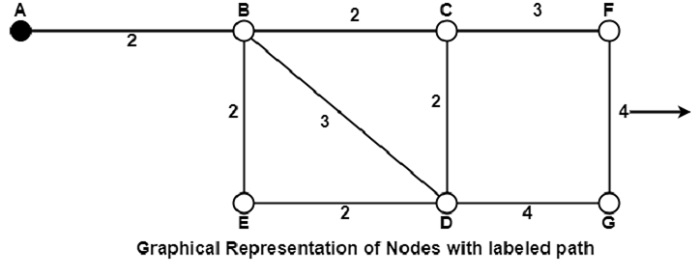# What is the Shortest Path Routing in Computer Network?

In this algorithm, to select a route, the algorithm discovers the shortest path between two nodes. It can use multiple hops, the geographical area in kilometres or labelling of arcs for measuring path length.

The labelling of arcs can be done with mean queuing, transmission delay for a standard test packet on an hourly basis, or computed as a function of bandwidth, average distance traffic, communication cost, mean queue length, measured delay or some other factors.

In shortest path routing, the topology communication network is defined using a directed weighted graph. The nodes in the graph define switching components and the directed arcs in the graph define communication connection between switching components. Each arc has a weight that defines the cost of sharing a packet between two nodes in a specific direction.

This cost is usually a positive value that can denote such factors as delay, throughput, error rate, financial costs, etc. A path between two nodes can go through various intermediary nodes and arcs. The goal of shortest path routing is to find a path between two nodes that has the lowest total cost, where the total cost of a path is the sum of arc costs in that path.

For example, Dijikstra uses the nodes labelling with its distance from the source node along the better-known route. Initially, all nodes are labelled with infinity, and as the algorithm proceeds, the label may change. The labelling graph is displayed in the figure.It can be done in various passes as follows, with A as the source.

• Pass 1. B (2, A), C(∞,−), F(∞,−), e(∞,−), d(∞,−), G 60

• Pass 2. B (2, A), C(4, B), D(5, B), E(4, B), F(∞,−),G(∞,−)

• Pass 3. B(2, A), C(4, B), D(5, B), E(4, B), F(7, C), G(9, D)

We can see that there can be two paths between A and G. One follows through ABCFG and the other through ABDG. The first one has a path length of 11, while the second one has 9. Hence, the second one, as G (9, D), is selected. Similarly, Node D has also three paths from A as ABD, ABCD and ABED. The first one has a path length of 5 rest two have 6. So, the first one is selected.

All nodes are searched in various passes, and finally, the routes with the shortest path lengths are made permanent, and the nodes of the path are used as a working node for the next round.